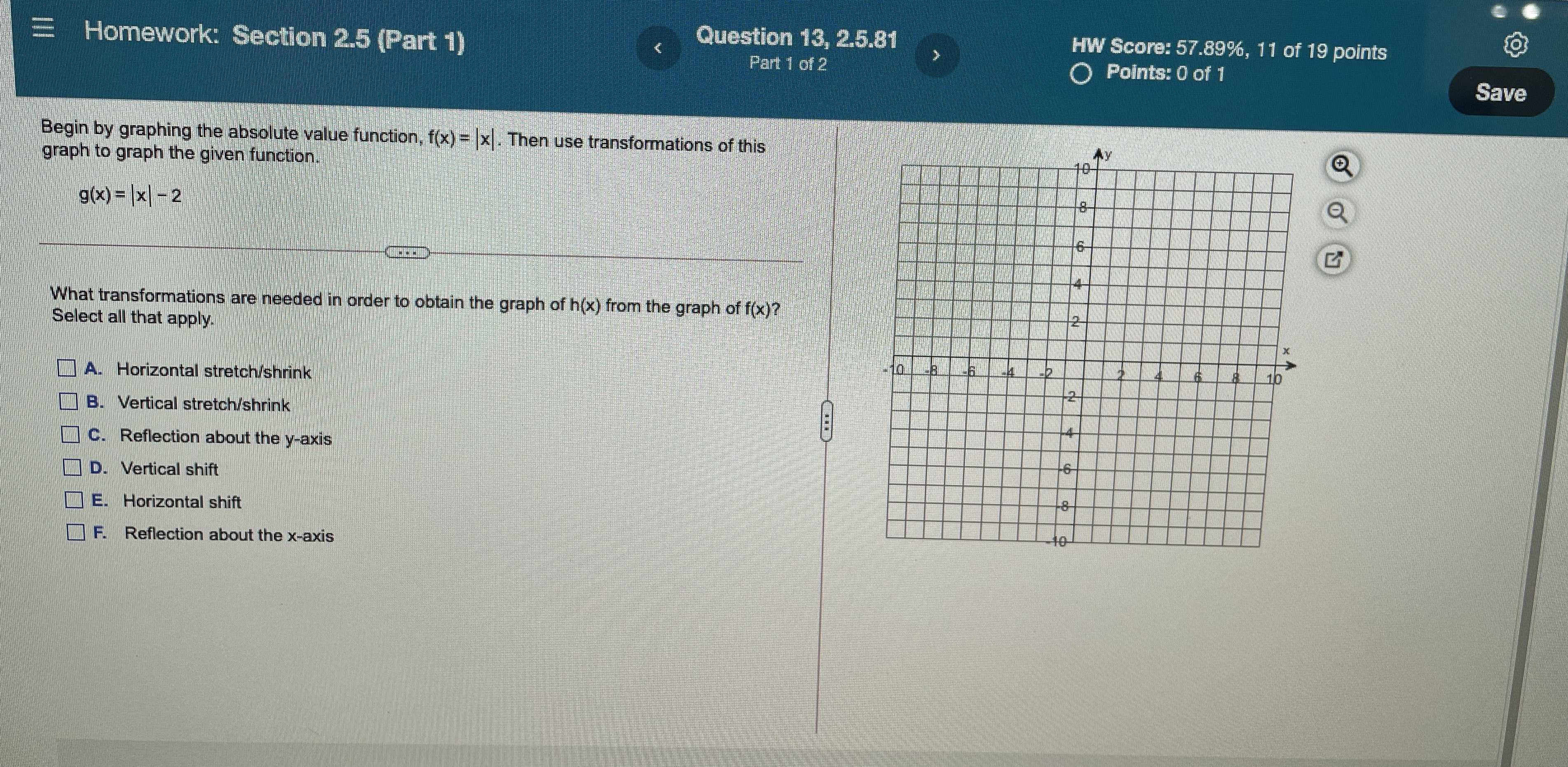### Still have math questions?

Algebra
QuestionBegin by graphing the absolute value function, $$f ( x ) = | x |$$ . Then use transformations of this graph to graph the given function.

$$g ( x ) = | x | - 2$$

What transformations are needed in order to obtain the graph of $$h ( x )$$ from the graph of $$f ( x ) ?$$

Select all that apply.

$$\square$$ A. Horizontal stretch/shrink

$$\square$$ B. Vertical stretch/shrink

$$\square$$ C. Reflection about the $$y$$ -axis

$$\square$$ D. Vertical shift

$$\square$$ E. Horizontal shift

$$\square$$ F. Reflection about the $$x$$ -axis